# Part 5 : Following along MIT intro to deep learningAbhijit Ramesh / March 28, 2021

12 min read––– views

## Introduction

### Learning in Dynamic Environments

So far we have seen deep learning where we are given data and the idea is to train a model around this data to do some prediction. In reinforcement learning, we have an agent which is placed in an environment and the agent is allowed to learn from that environment by exploring and interacting with this environment. They do this without any form of human supervision.

Reinforcement learning is used in fields such as robotics, gameplay and strategy. It bridges the gap between the virtual worlds of deep learning and the real world or robotics.

## Classes of Learning problems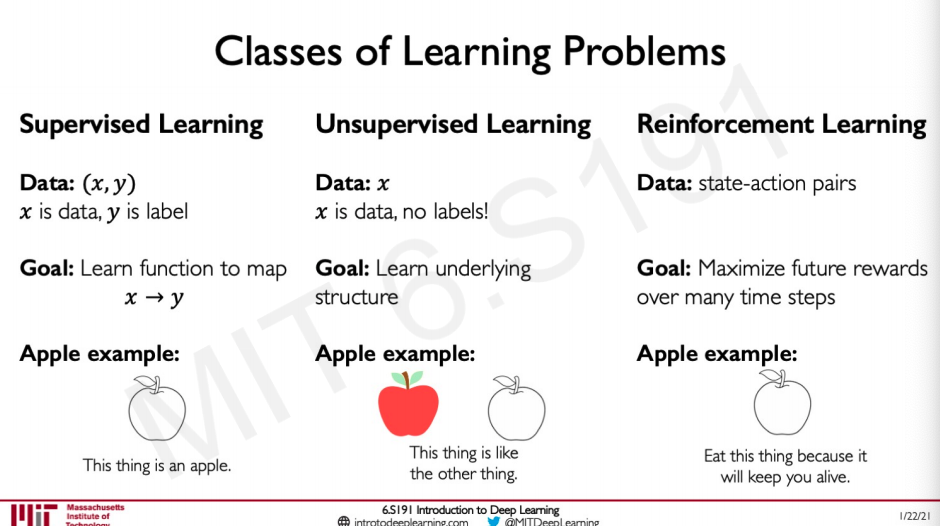We have been mostly dealing with supervised learning were we are given a data x and the label y and our job is to train a model that does the mapping from x to y by learning the function.

Another form of learning is unsupervised learning where there is only data and no labels the model is trained to do some form of clustering to recognise that if something is an apple or or not by learning from the similarity or dissimilarity of these data points.

Now we will be looking at reinforcement learning where there is some established state-action pair and the goal is to maximise the rewards based on the actions the agent takes over many time steps. So this would be something completely different from the other two examples like eat this apple to stay alive, the model might have learned that eating the apple allowed the agent to stay alive for a longer period so now it knows how to stay alive by eating this apple.

## Definitions

#### Agent

The algorithms that learns is called the agent, in the Reinforcement learning words this is the algorithm in real world this can be us.

#### Environment

The word where the agent exists and operates

#### Actions

A move that an agent can make in the environment $a_t$

#### Action space A

Set of possible actions an agent can make in the environment, this can be discrete or continuous based on the environment and the problem we are solving.

#### Observations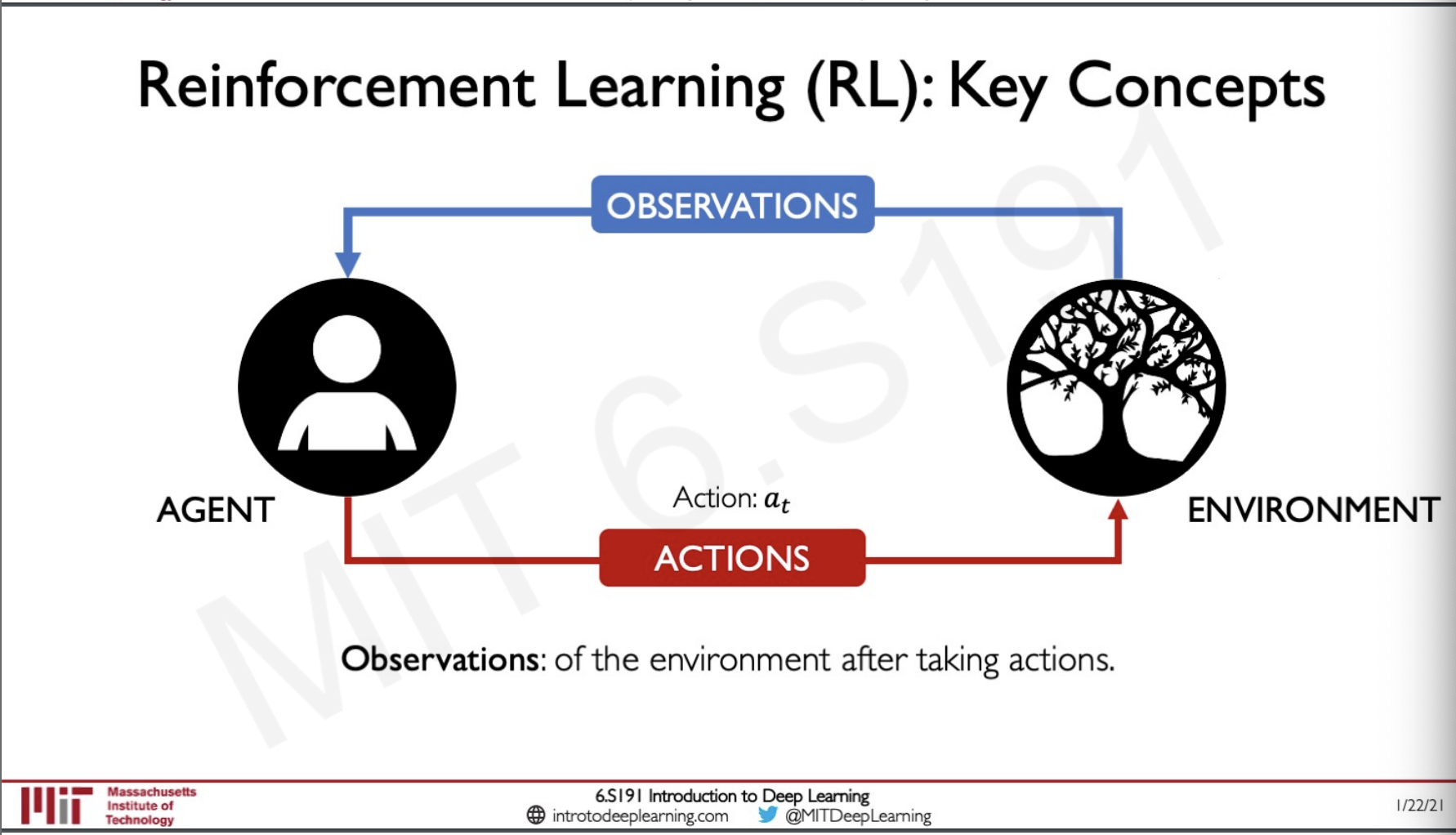The observation of the environment after the agent has taken an action.

#### State

A situation where the agent has been put in after an action has been taken and the agent perceives it. $s_\bgroup t+1\egroup$

#### Reward

The feedback the agent receives after measuring success of failure of the agent's action. $r_t$

#### Total Reward

$R_t= \sum_\bgroup i=t\egroup^\bgroup \infty\egroup r_i$

The total reward the agent earns over time period t.

#### Discounted Reward

$R_t= \sum_\bgroup i=t\egroup^\bgroup \infty \egroup \gamma^i r_i$

The total reward the agent earns over time period t discounted by a factor of $\gamma$ this is so that the agent can be more attracted to short term reward than long term reward.

## the Q-function

The q function is used to capture the expected total future reward an agent in a state $s$ can receive by executing a certain action $a$

$Q(s_t,a_t) = E[R_t|s_t,a_t]$

So how does the agent take an action given this magical function that predicts the future reward?

Well the strategy is to choose an action that maximises future reward.

$\pi^*(s) = argmax_aQ(s,a)$

There are two methods to do deep reinforcement learning,

### Value Learning

This is to find the Q function that is $a = argmax_aQ(s,a)$

### Policy Learning

This is to learn the policy $\pi(s)$ itself rather than using the Q function and then Sample $a \sim \pi(s)$

## Digging deeper into the Q-function

For humans, it would be very difficult to estimate the Q-function for example,

Let us consider the Atari breakout game where there are some blocks, a paddle and a ball. The objective of the game is to make the paddle go left , right or be in the same position in such a way that the blocks get hit by the ball and breaks the game finishes. The agent here is the paddle , the actions it can take is to move left, right or be stable. The reward is breaking a block.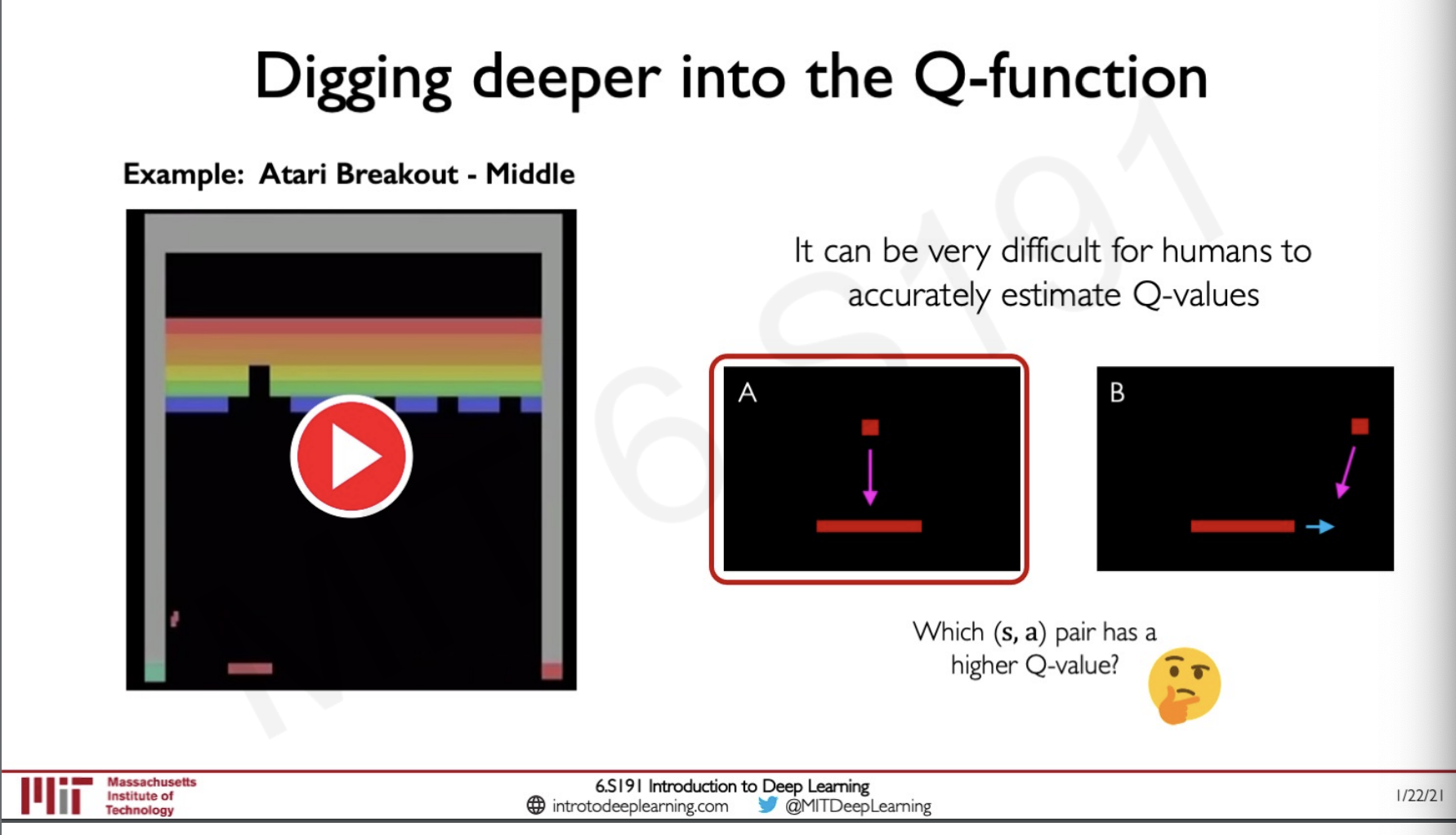Here there are two Q functions a weather to position the paddle in such a way that the ball will fall right into it or b weather to hit the ball with the side of the paddle so that the ball bounces in drastic angles.

Here in this video from [0:00] to [0:43], the agent is following the first policy where even though it is playing the game well it is not doing it fast, eventually, the agent starts using policy b and it figures out that if it breaks the coroners first and then allows the ball to reach on top the blocks get broken without having to take any actions here the policy is actually maximising the reward with very few actions and we can say the agent has learned the best policy. Even though the policy can be easily determined by an agent it is hard for a human to figure out.

## Deep Q Networks

So now the question is how do we use these deep Q networks ?

As we told before it is hard for a human to define these Q functions so we can use a neural network to learn the function and then use this in the game. To model this function we need to take in input as the state and the action and the agent learns the Q value and gives it as output.

This does not really give us a very good intuition into how the network is modelling the states so we need to dig deeper. We are not sure that the Q value given by the network is the best rewarding function so the way of solving this is by predicting a Q value for all the possible set of actions.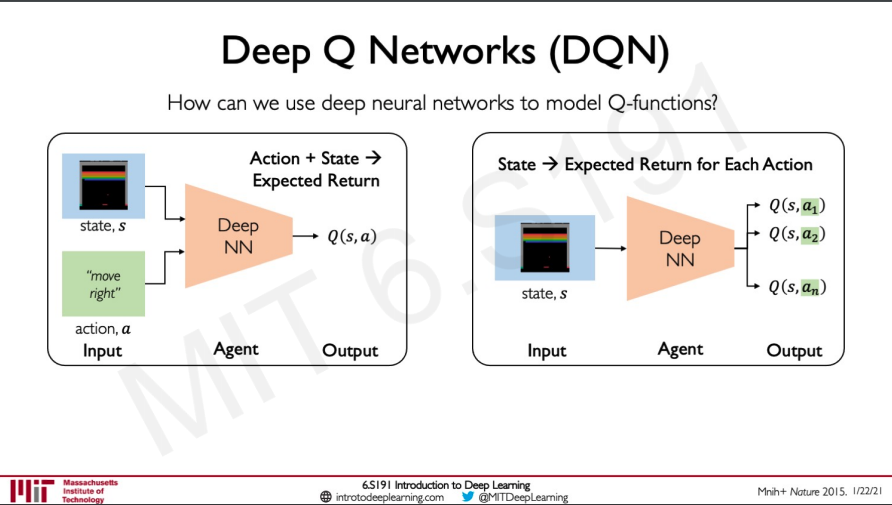We do not have a data saying what are the Q values so training the model means taking all the best actions.

The target return for taking all the best action would be

$(r+ \gamma max_\bgroup a' \egroup Q(s',a'))$

Now that we have a target Q value and a predicted Q value we can easily compute a loss called Q-loss

$L = E[||(r+ \gamma max_\bgroup a' \egroup Q(s',a')) - Q(s,a)||^2]$

So putting it all together,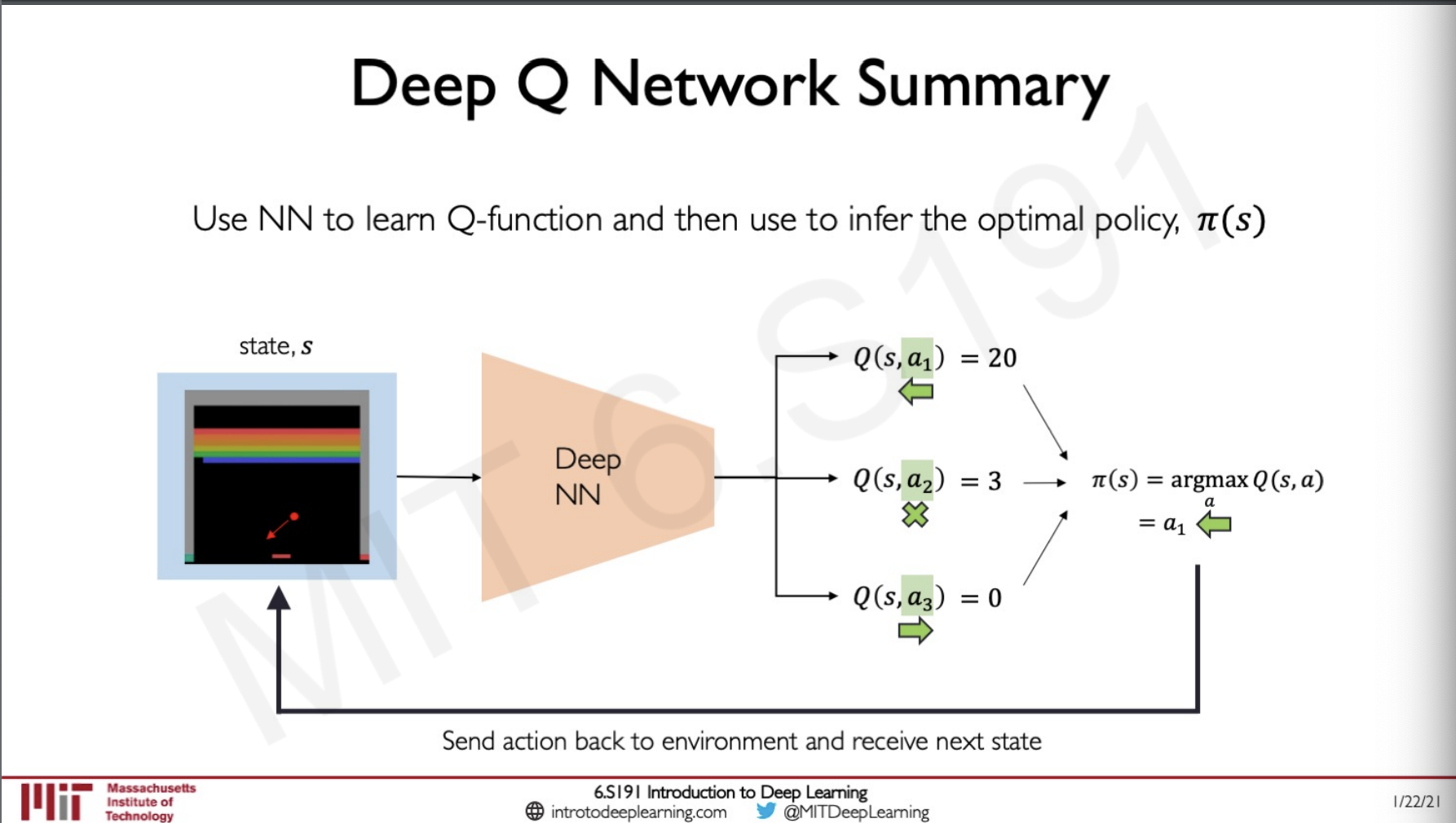The goal of the model is to maximise the reward for the given policy, so it would compute the Q value for all the possible actions in a particular state it can take and the best one from it to find the best Q-function.

## DQN Atari Results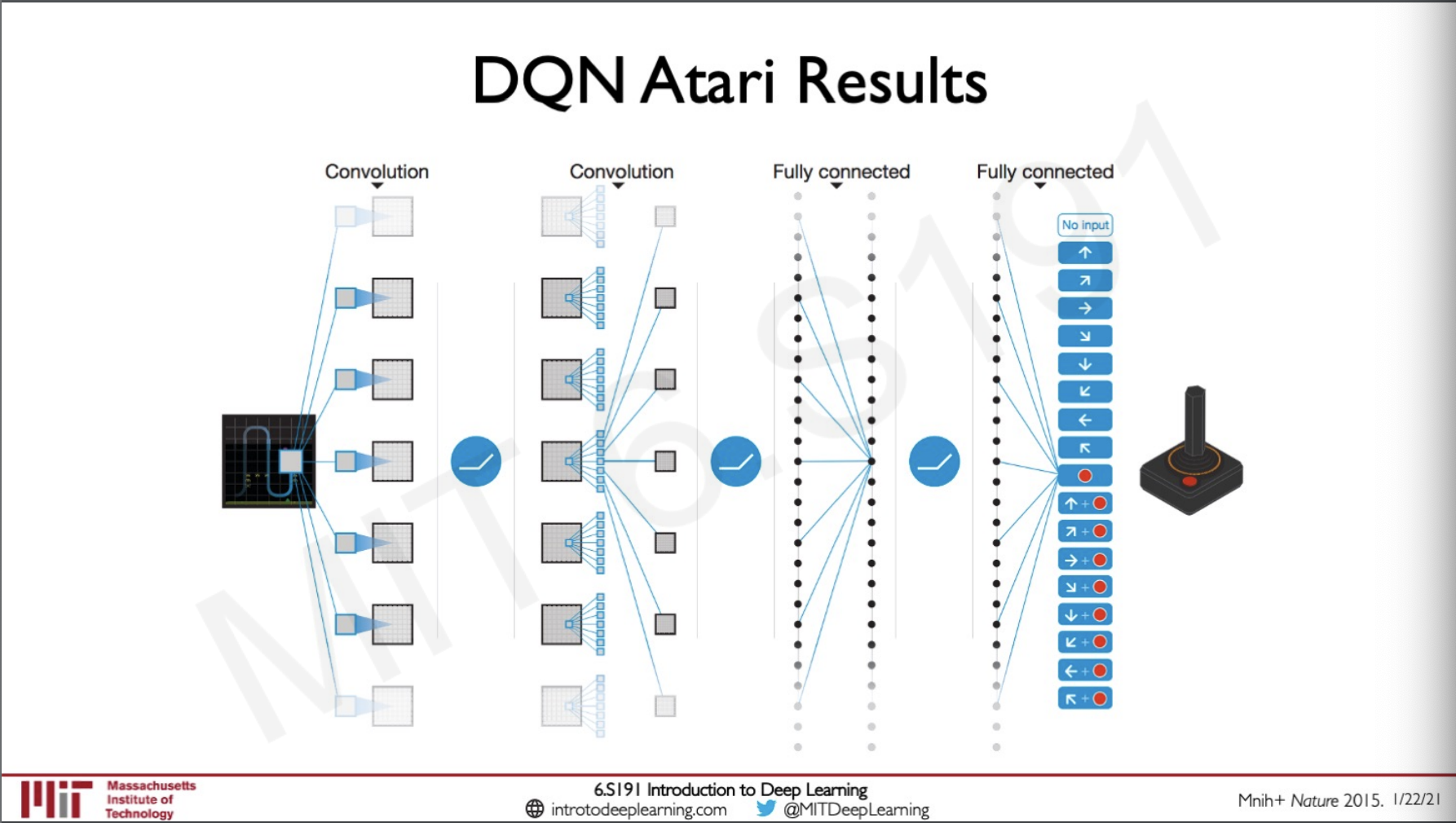A similar DQN as we have seen earlier was used in many other Atari games as well not only breakout this was done by feeding the model inputs as the images through convoluted and fully connected layers and then using this to predict a Q function.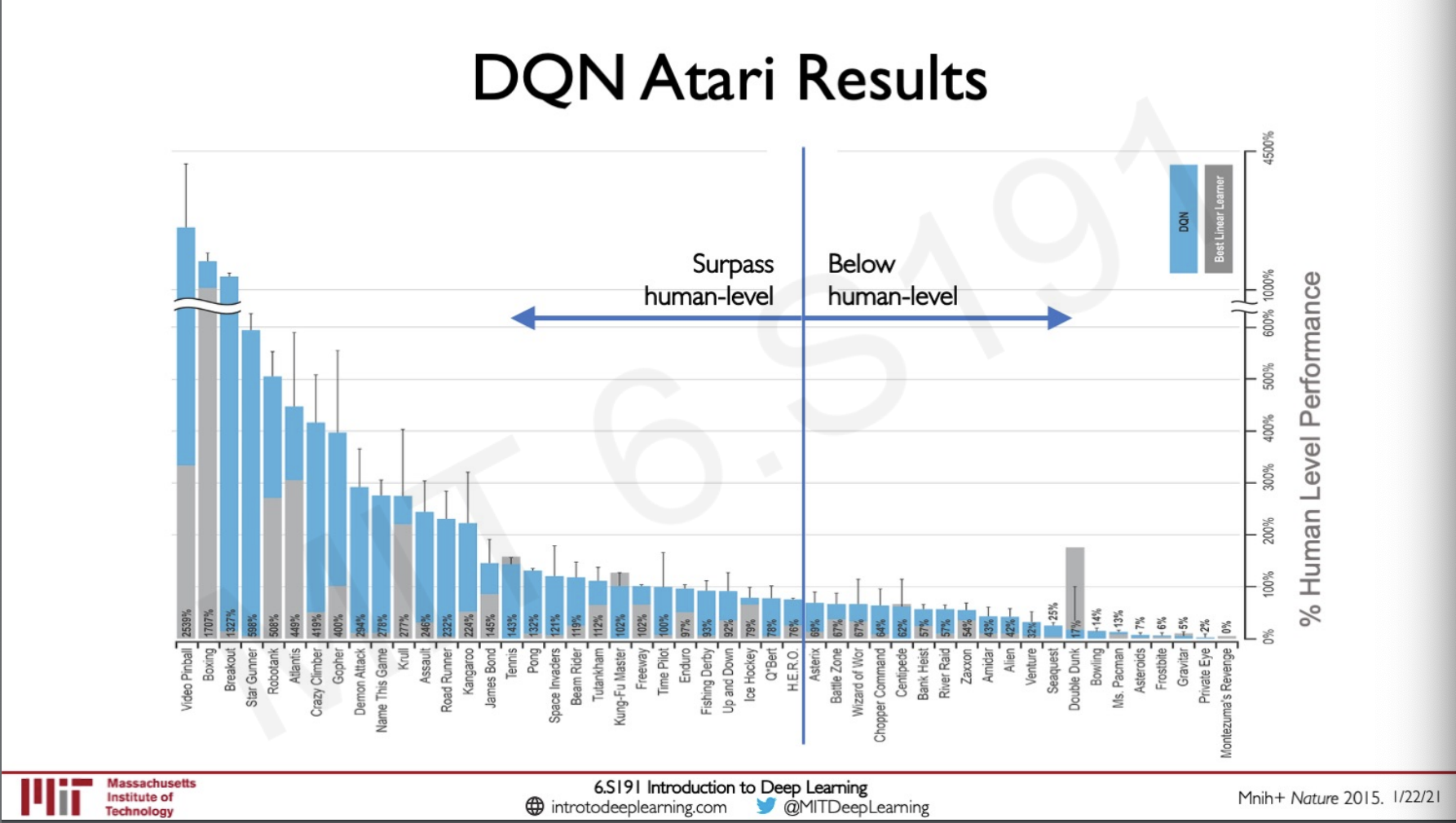This method actually surpassed human level performance in most of the games, this is actually a very surprising considering how straight forward the technique is and it was able to be applied universally to many games.

## Downside of Q-learning

All these actions we have seen so far are discrete that is the action space is divided into bins and this value learning method can only be applied in these case, if there is a continuous action space this model is not able to handle it.

The learning process is not stochastic it is deterministically computed from Q function by maximising the reward.

This can be addressed by considering Policy Learning apporches

## Policy Learning

Instead of finding the Q function and then using that to predict what policy we should take in policy learning as the name suggest we will be learning the policy directly.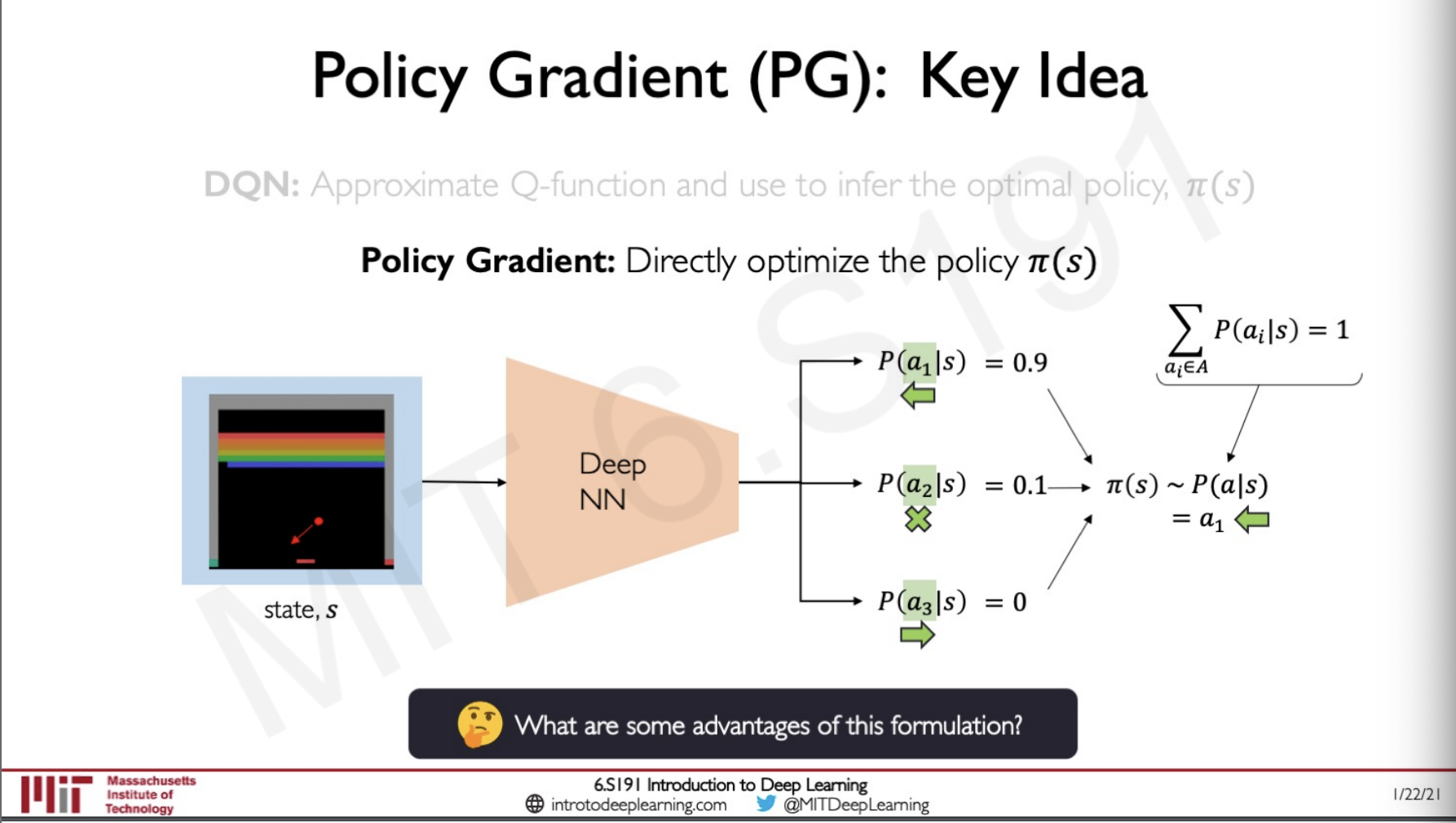Here we are directly optimising the policy $\pi(s)$ so what happens is that we will be giving action to be taken and would get a function that describes the probability distribution from which we can sample to know which action would have the probability of giving the best reward and therefor is the best policy.

## Advantages of Policy Learning

### Discrete vs Continuous Action Spaces

A discrete action space is where we are given a particular set of direction and the probability of which one of this we should be taking would be higher but if we consider something like lets say the velocity in which the the paddle should be moving this would become continuous, to move left this would be negative and to the right would be positive, we can also visualise this distribution by plotting this probability distribution.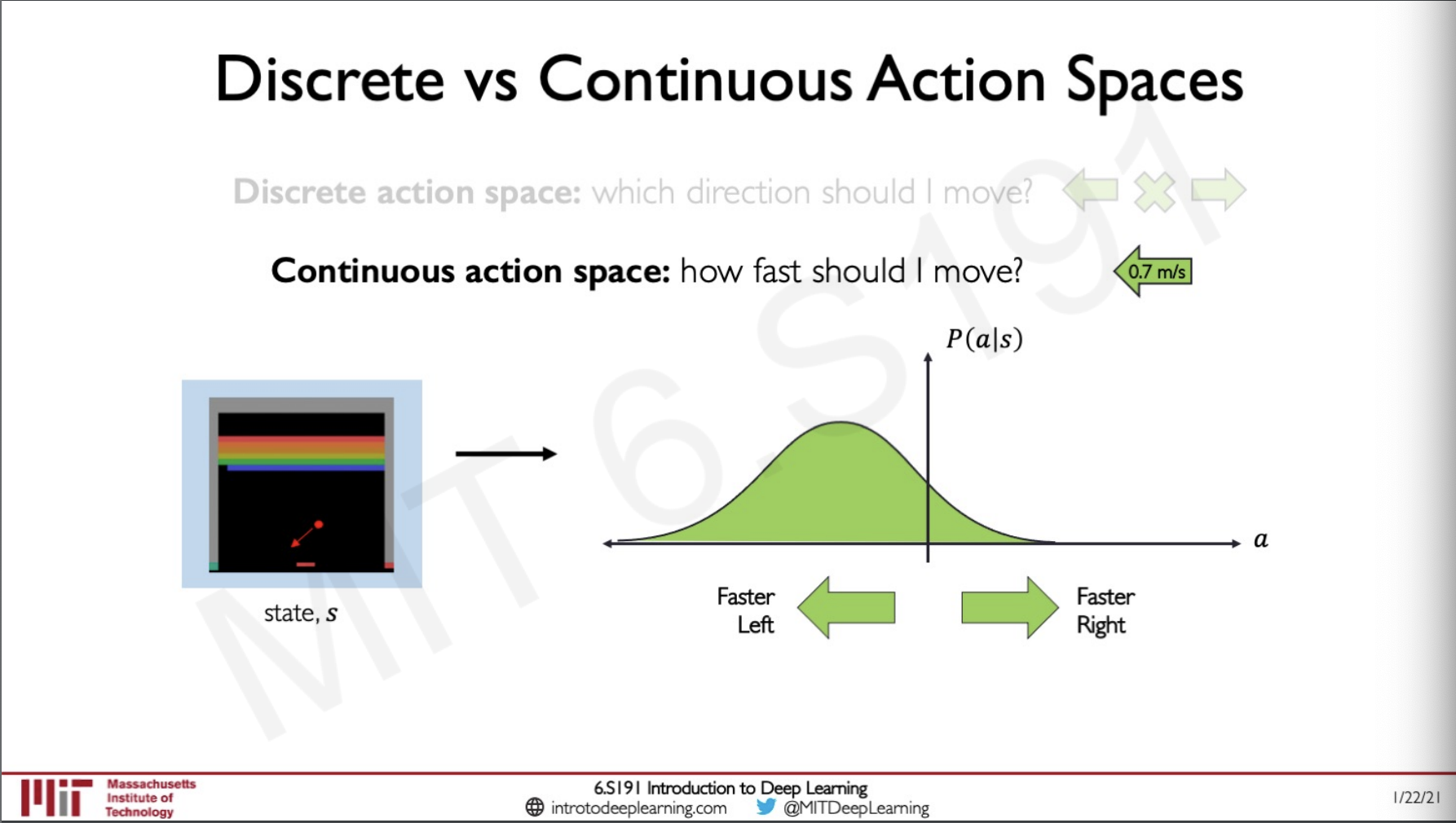Since the outputs are continuous instead of predicting in a infinite space lets say we output to a normal gaussian distribution.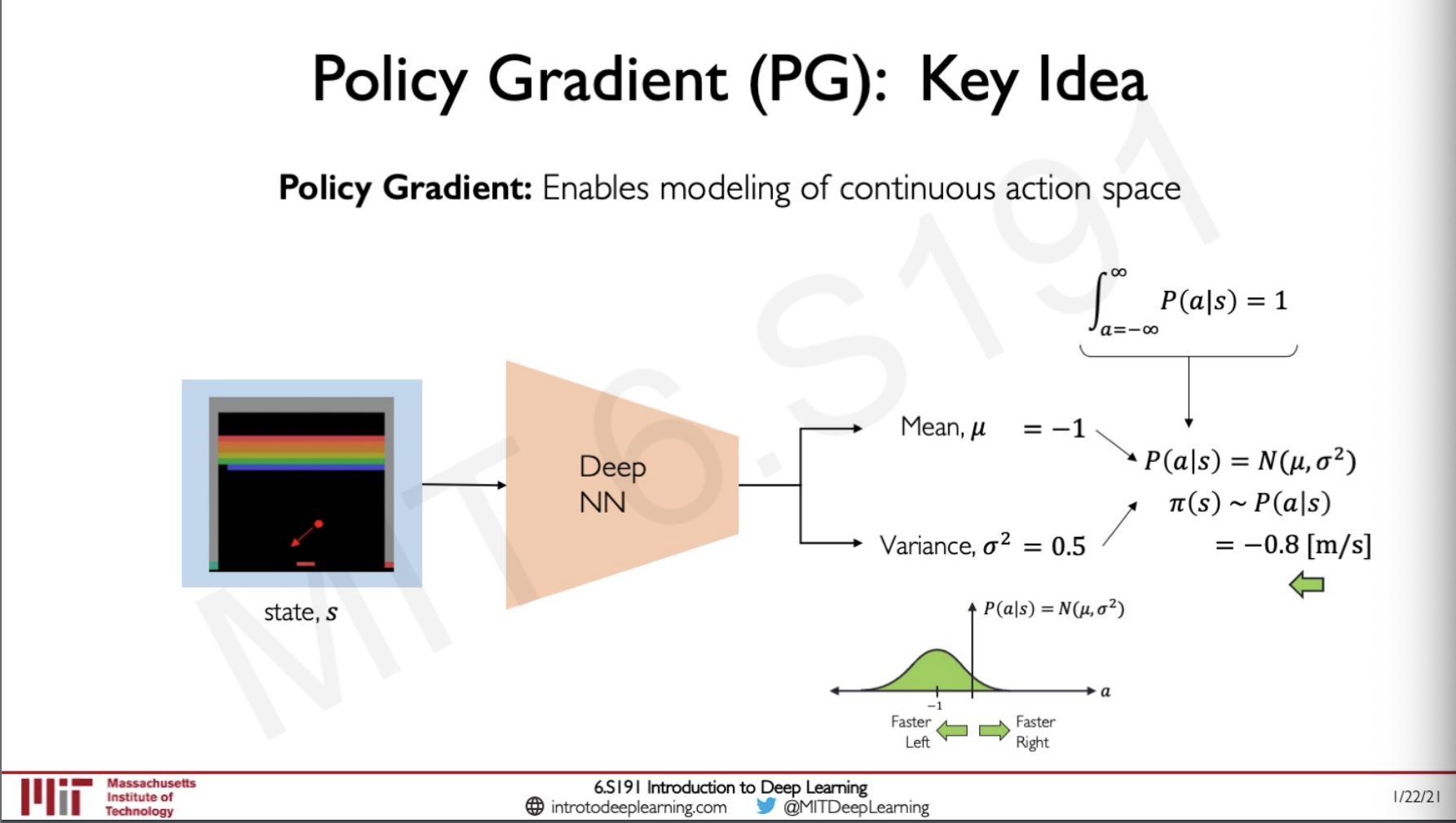So the output would be two values a mean and a variance, and we could plot this in distribution and figure out what action is to be taken by finding the maximum point in the plot here we can see that with a mean of -1 and variance of 0.5 the policy is giving the output to be -0.8[m/s] that is 0.8 m/s to the left.

We can also integrate the policy distribution from -infinity to infinity to get the result as one, this property can be used as a verification if what we are doing is correct.

## Training Policy Gradient : Case Study

Let us consider an example of a self driving car,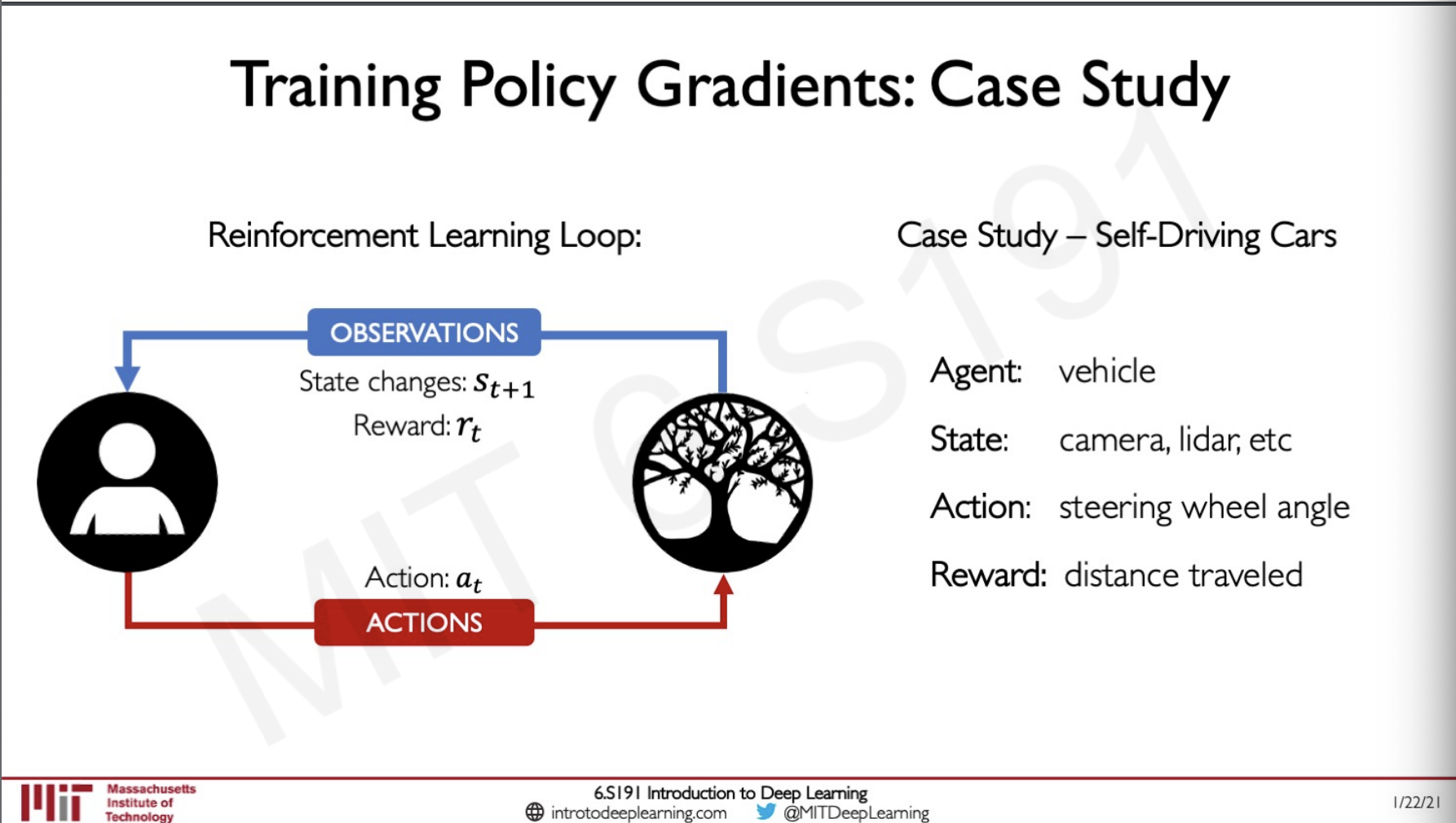Here we have defined our agent, state, action and reward.

Our training algorithm has four steps

1. Initialise the agent
2. Run a policy until termination
3. Record all states, actions, reward
4. Decrease the probability of action that result in low reward
5. Increase the probability of action that result in high reward

For the drive-less car, let's say we are running this algorithm, and it took 5 steps in the first iteration and the car crashed because we have not trained our policy yet so now the algorithm is in step 4 and it would try to reduce the probability for the last 3 steps the car took and would increase the first two steps the car took since this may not be the reason for the car to crash. The last part the algorithm did was not very Intelligent because we are not sure if this is any benefit for the algorithm to do but this leads to simplicity because the RL algorithm has no intrusion on what a car is or a lane is but it learns how to drive anyways by maximising not step 4 and 5.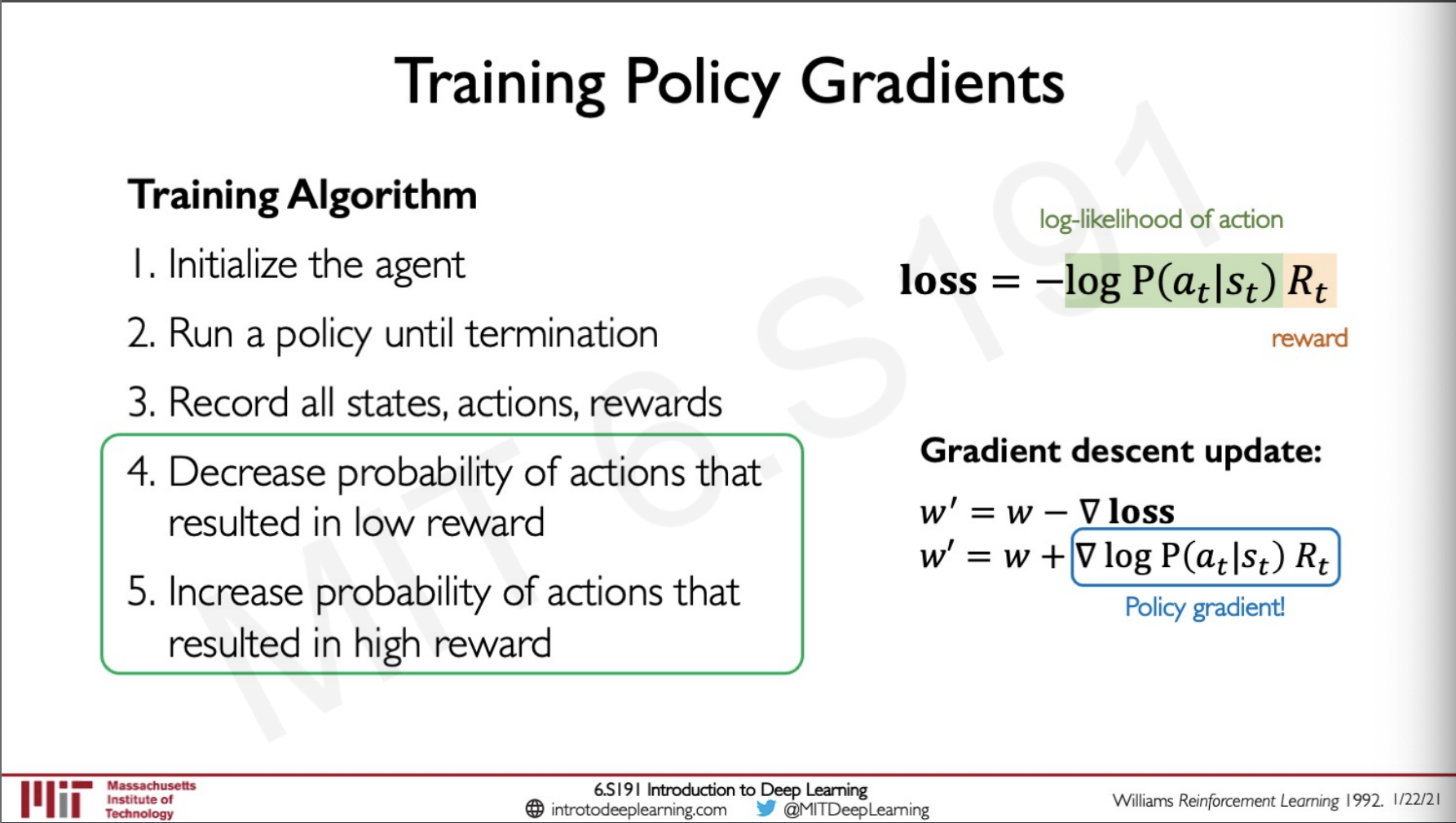The loss here has two components the log-likelihood of an action and the reward, depending on the probability of the action the reward would be amplified and if the action gives a very less reward here the loss is made to understand not to follow that action the next time, we can plug in this value inside the gradient descent algorithm and thus allow to maximise step 4 and step 5 and train the network.

## Training in Real Life

The problem with training a RL algorithm in real life is our step 2 of the algorithm, that is Run a policy until termination what this means is that termination in real life might be crashing or dying or something bad like that which we do not want to happen so one way to do this is to simulate the world and then try running the algorithm there but this may not result is very accurate results transferring to the real world.

The video shows a demo of VISTA a simulator build at MIT by Alexander Amini and the team which gives a photorealistic simulation to train reinforcement learning self-driving cars that is very representative of the real world.

## Deep Reinforcement Learning Applications

One of the most famous application go RL we all know would be playing the game of go the challenge with this game is that the number of possible states in this game is more than the number of atoms in the universes, Googles alpha go was one of the models that was first to beat grandmasters in the game of go.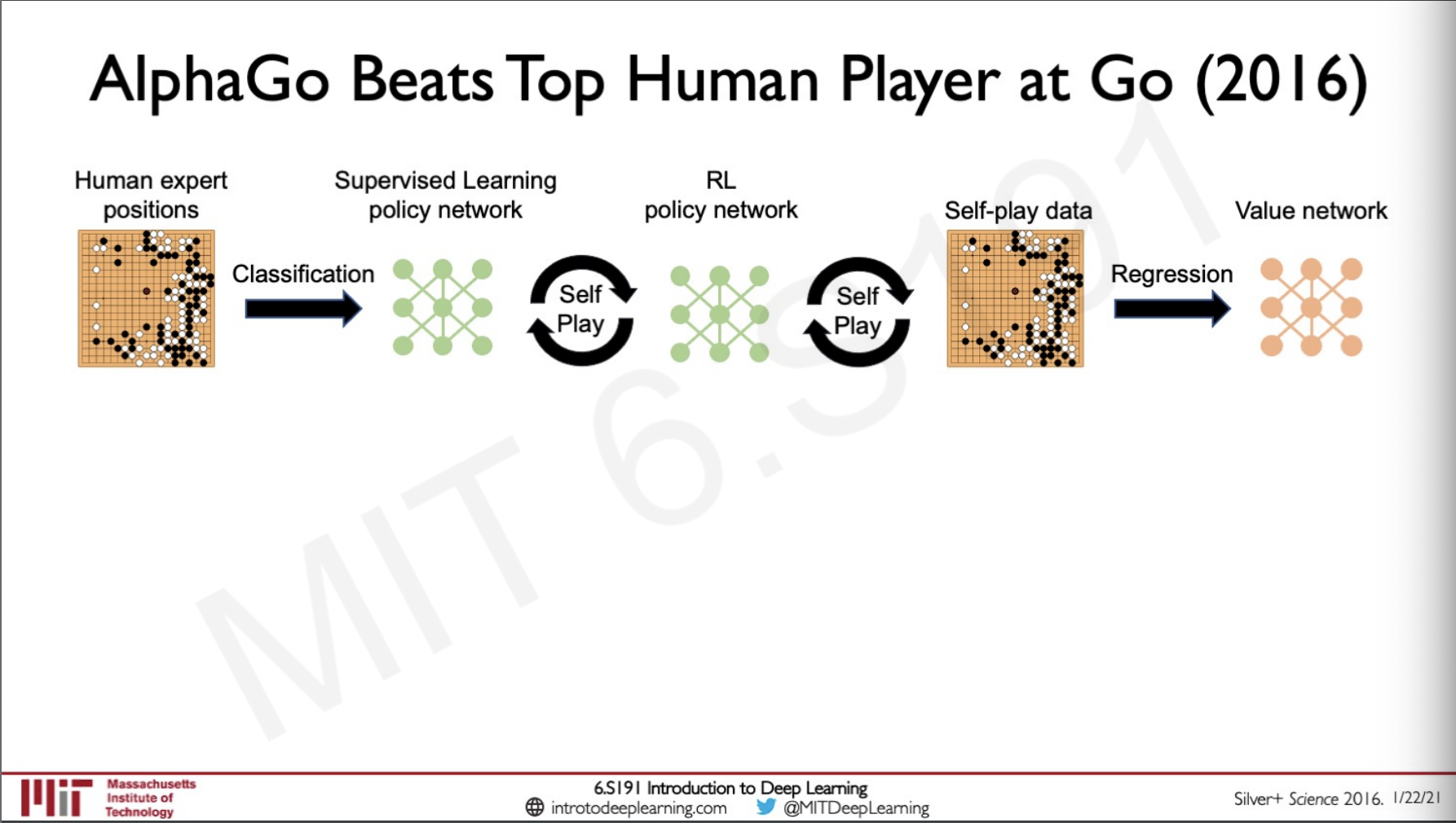Alpha go was initially train by supervision where the model observed games by experts and then used this network to then do Reinforcement learning by self playing to get better results than grandmasters, the self play data was further used for regression to create a value network that had an intuition of the board states.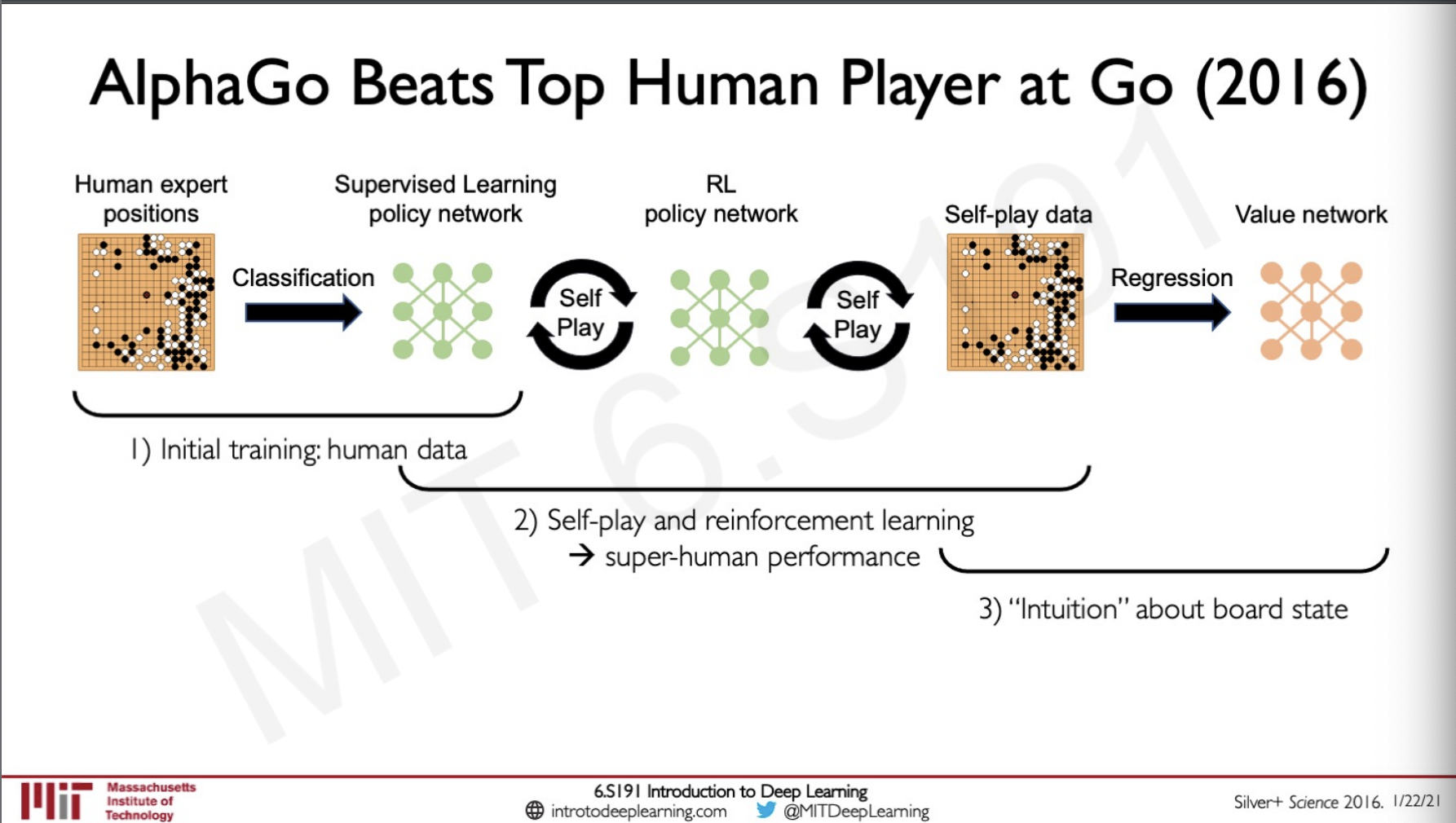After alpha go, alpha zero was created which was completely learned on self play and was good at other games as well like go, chess and shogi.

Recently google developed MuZero which was not given any kinds of knowledge about the rules of the game and was asked to learn from it this algorithm was expert in not only go, chess and shogi but also in atari games.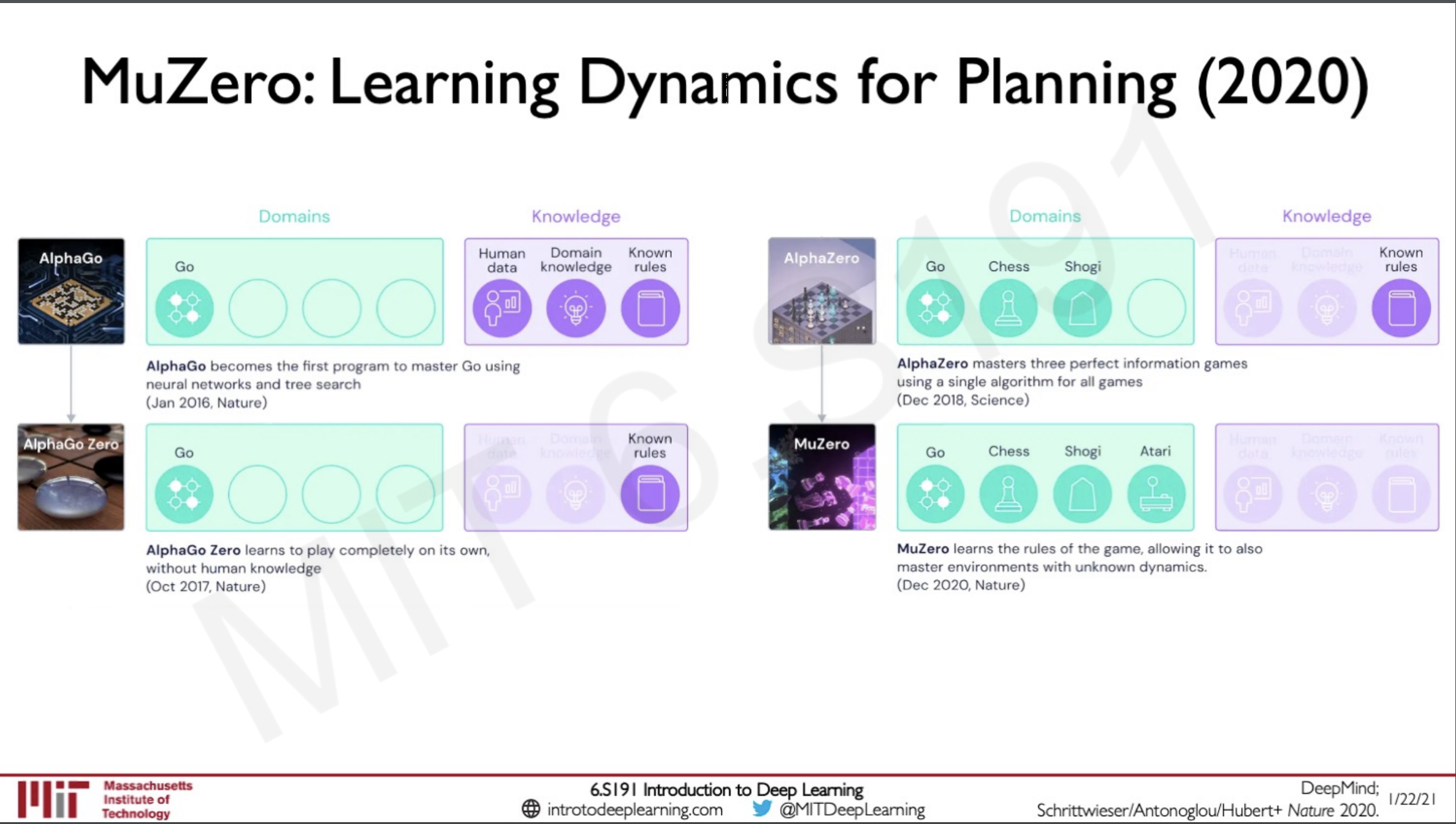Muzero algorithm works in four states

1. Observe
2. Search
3. Plan and
4. Act

First, it observes all the available states in the game and then creates a searchable tree from which to represent the possible scenarios that can arise. Since we do not know the rules the network is made to learn the dynamics of how to do this search. This would give us the probability of the actions it can take from which the model can plan what action to take next until the game finishes.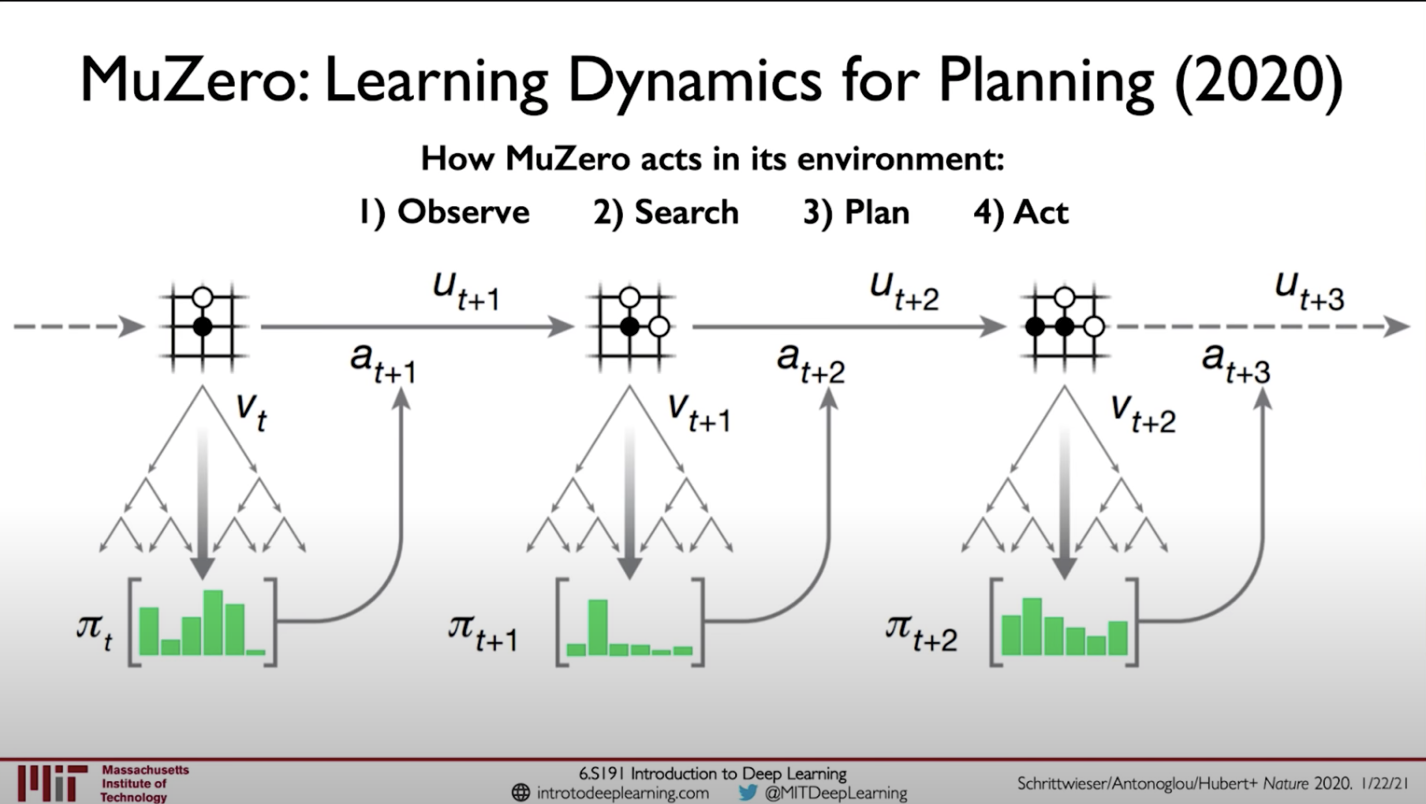## Sources

MIT intro deep learning : http://introtodeeplearning.com/

Slides on intro to deep learning by MIT : http://introtodeeplearning.com/slides/6S191_MIT_DeepLearning_L5.pdf

## Lab Solutions

https://github.com/abhijitramesh/learning-introtodeeplearning/blob/main/lab3/RL.ipynb

##### Subscribe to the newsletter

Get emails from me about machine learning, tech, statups and more.

- subscribers